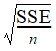# NMath User's Guide

38.7 Spread (.NET, C#, CSharp, VB, Visual Basic, F#)

Measures of spread are measures of the degree values in the data set differ from each other. For example, the static SumOfSquaredErrors() method on class StatsFunctions calculates the sum of squared errors (SSE) of the elements in the data set. SSE is the sum of the squared differences between each element and the mean.

StandardDeviation() computes the biased standard deviation of the elements in a data set.For instance:

Code Example – C#

```double stdev = StatsFunctions.StandardDeviation( data );
```

Code Example – VB

```Dim StdDev As Double = StatsFunctions.StandardDeviation(MyData)
```

Alternatively, you can specify the unbiased standard deviationusing a value from the enumeration:

Code Example – C#

```double stdev =
```
```  StatsFunctions.StandardDeviation( data, BiasType.Unbiased );
```

Code Example – VB

```Dim StdDev As Double = StatsFunctions.StandardDeviation(MyData,
BiasType.Unbiased)
```

NOTE—StatsSettings.Bias specifies the default BiasType.

Variance() calculates the variance of the elements in a data set. Variance is the square of the standard deviation. Again, you can specify a biased or unbiased estimator using values from the enumeration.

MeanDeviation() calculates the mean deviation of the elements in a data set. The mean deviation is the mean of the absolute deviations about the mean. The mean deviation is defined bySimilarly, MedianDeviationFromMean() calculates the median of the absolute deviations from the mean. MedianDeviationFromMedian() calculates the median of the absolute deviations from the median.

Lastly, InterquartileRange() returns the difference between the median of the highest half and the median of the lowest half of the elements in a data set:

Code Example – C#

```double iqr = StatsFunctions.InterQuartileRange( data );
```

Code Example – VB

```Dim IQR As Double = StatsFunctions.InterquartileRange(MyData)
```

Top

Top